Open main menu
SUPPORT DOCUMENTATION

### Cross-axis rejection

Cross-axis rejection is the ability of a component of a triaxial instrument to ignore signals which arrive perpendicular to the axis of sensitivity of that component. It is normally expressed in deciBels and a larger number signifies better performance. It can be thought of as the amount of signal leakage between components: a purely vertical excitation should not generate any signal on the horizontal outputs, for example. Any signal which does appear is unwanted and problematic. In practise, this leakage can be minimised but never totally eliminated.

The diagram below shows a top-down view of the two horizontal components of an ideal triaxial instrument. The green signal represents ground motion arriving perfectly perpendicular to one of the components. The ground movement is indicated by a symbolic sine-wave but the actual movement is in the direction of the red arrow and back (i.e. in the left⇔right direction in the first diagram). The masses are represented by the grey areas in the diagram.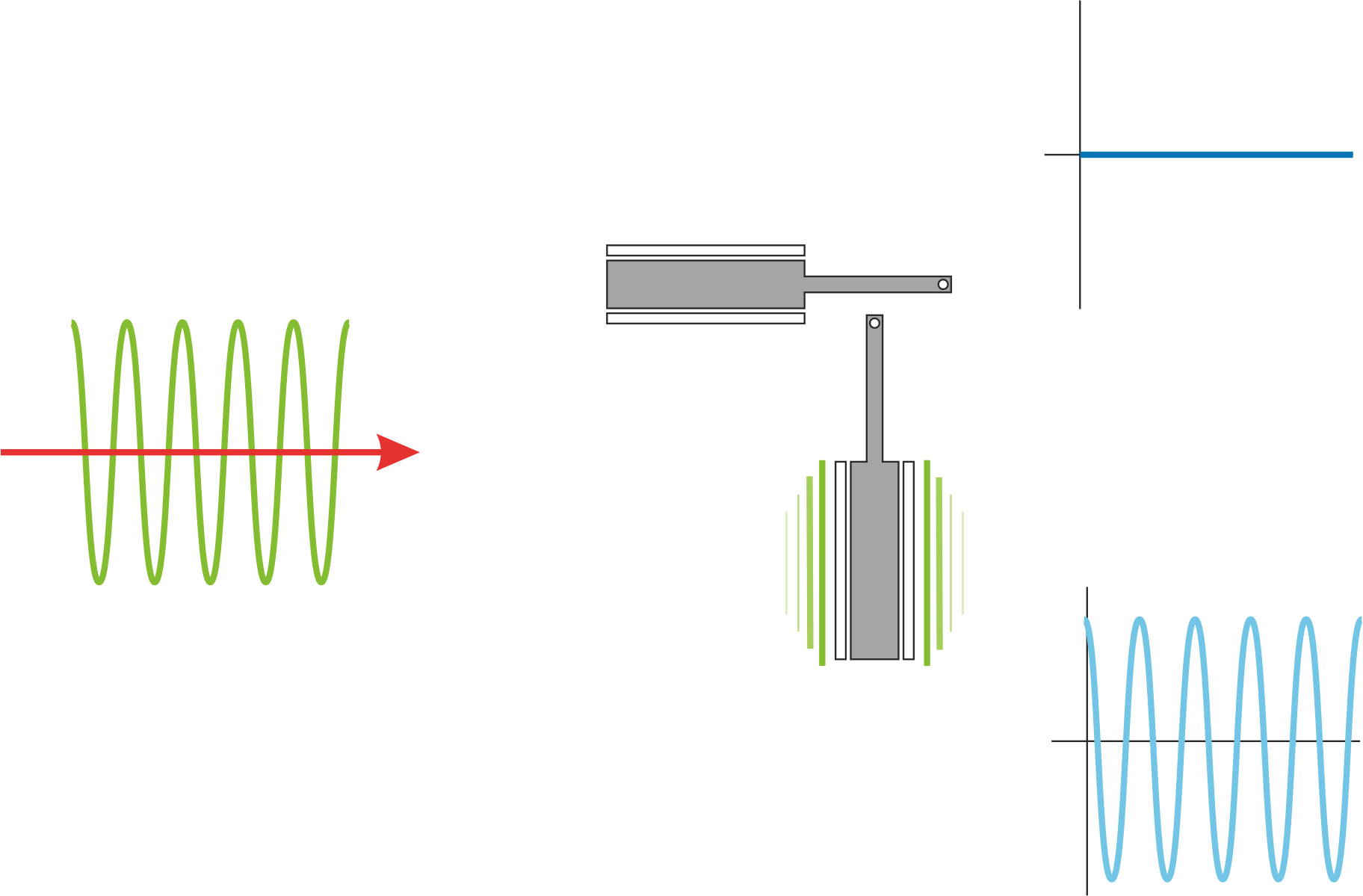It is clear that, for an ideal instrument, the output signal from the component perpendicular to the incoming signal should be strong while the output signal from the component parallel to the direction of the incoming signal should be zero. The two output signals are represented in the diagram by the blue traces.

If the same signal were to arrive from a direction perpendicular to the original direction, the outputs would be as shown below.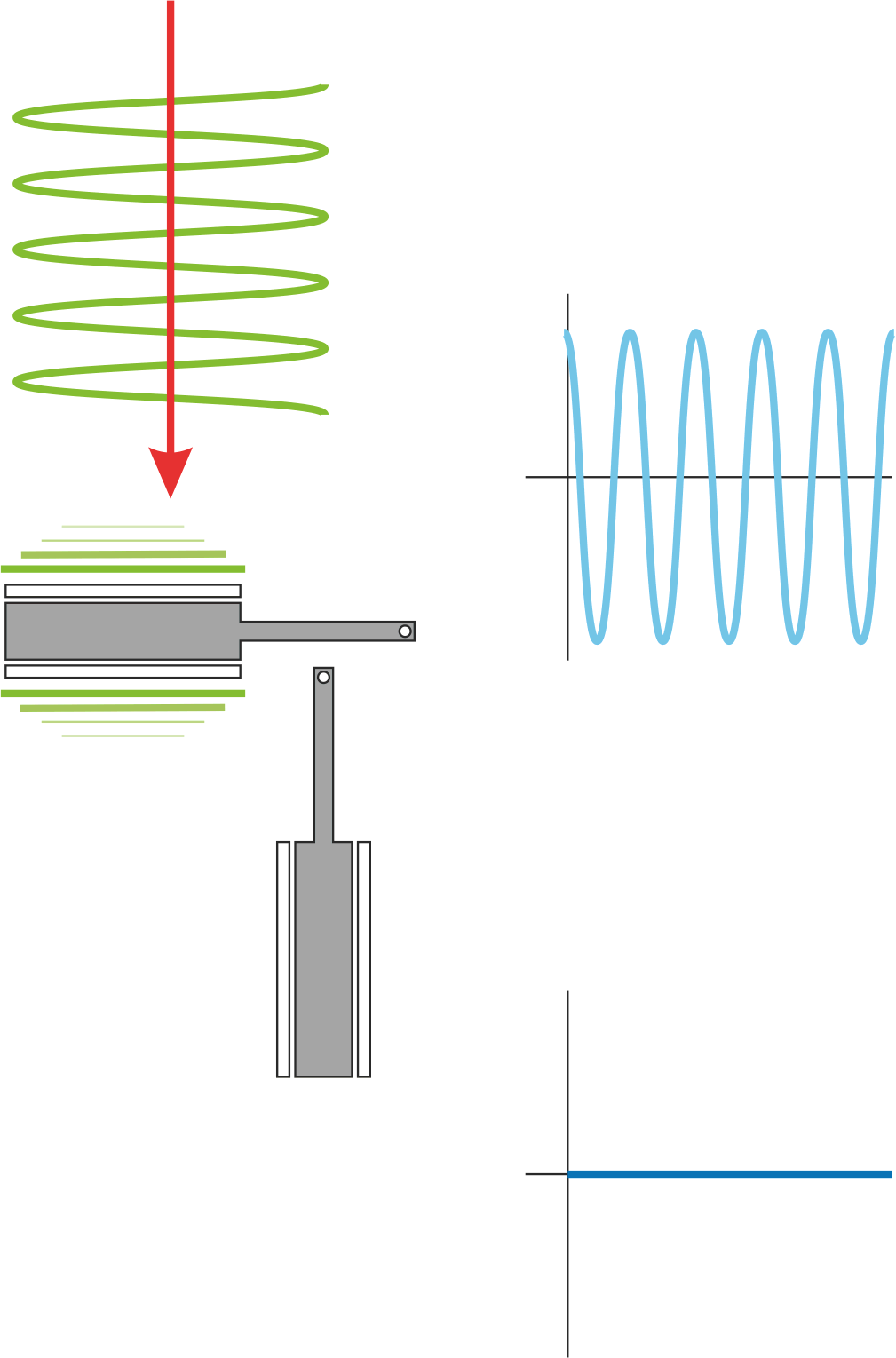Again, one signal is strong while the other is zero.

If the incoming signal were to arrive at 45°, the outputs from the two components would be equal at around 71% of the original, maximum amplitude.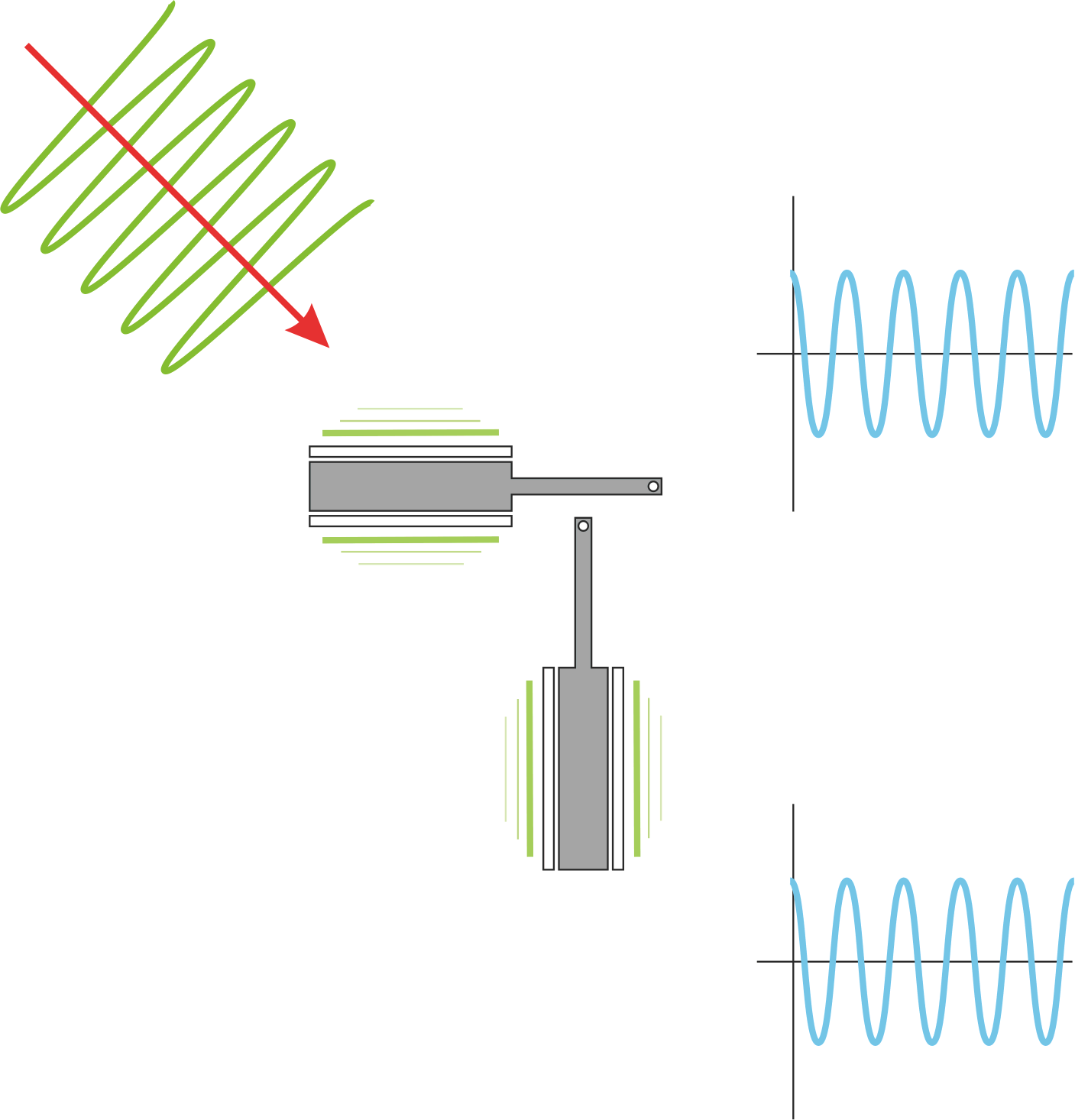Now consider an instrument where the two components are not mechanically aligned perfectly. If the incoming signal is parallel to one of the components, that component will still show zero output. The other component is no longer perpendicular to the incoming signal, so the output amplitude will be decreased slightly compared to the expected level. This is a problem but it would be hard to measure in practice.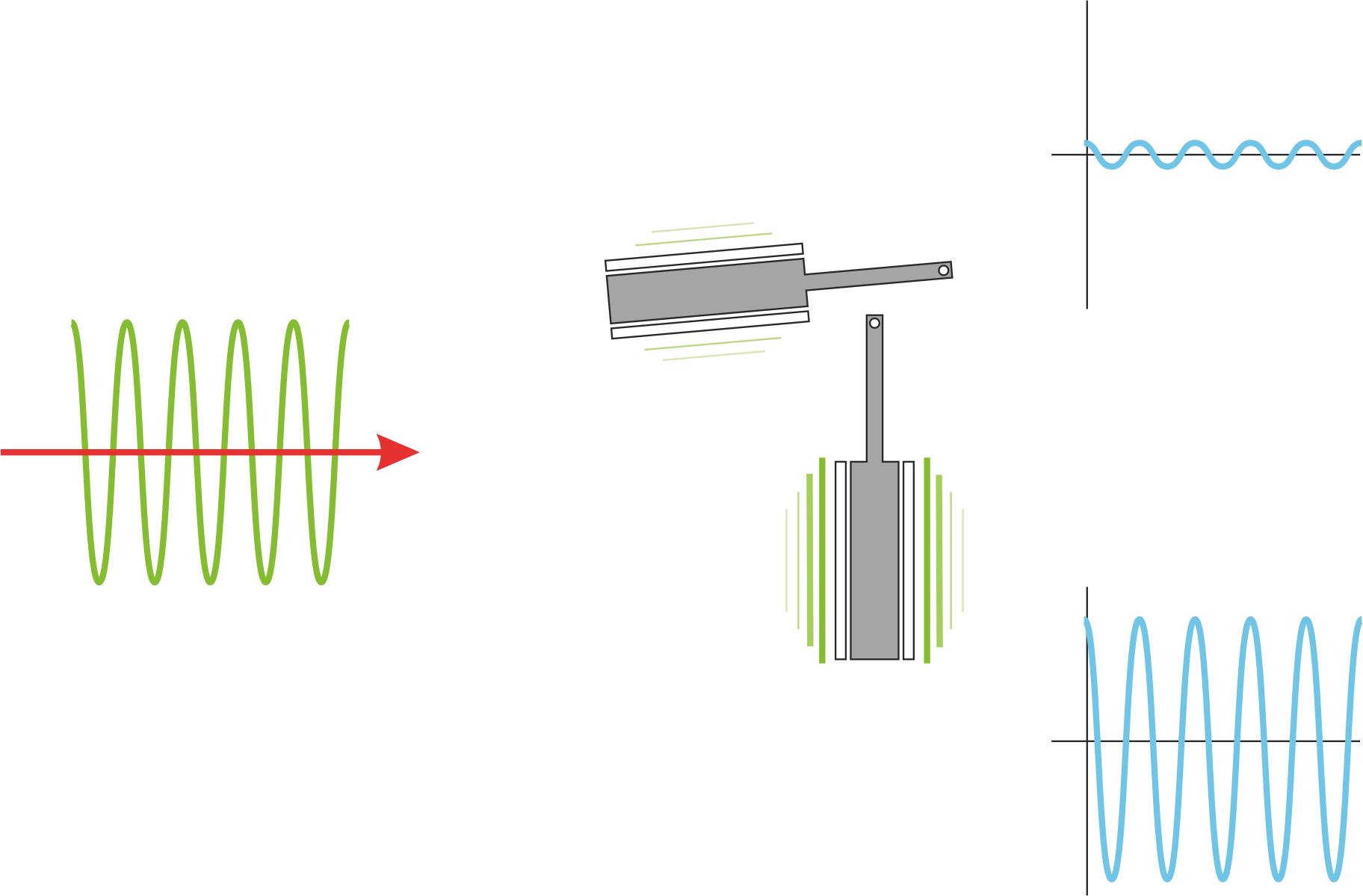If the input signal arrives perpendicular to one component, however, an error signal will appear on the other component, where we would expect the signal to be zero. This is easier to measure.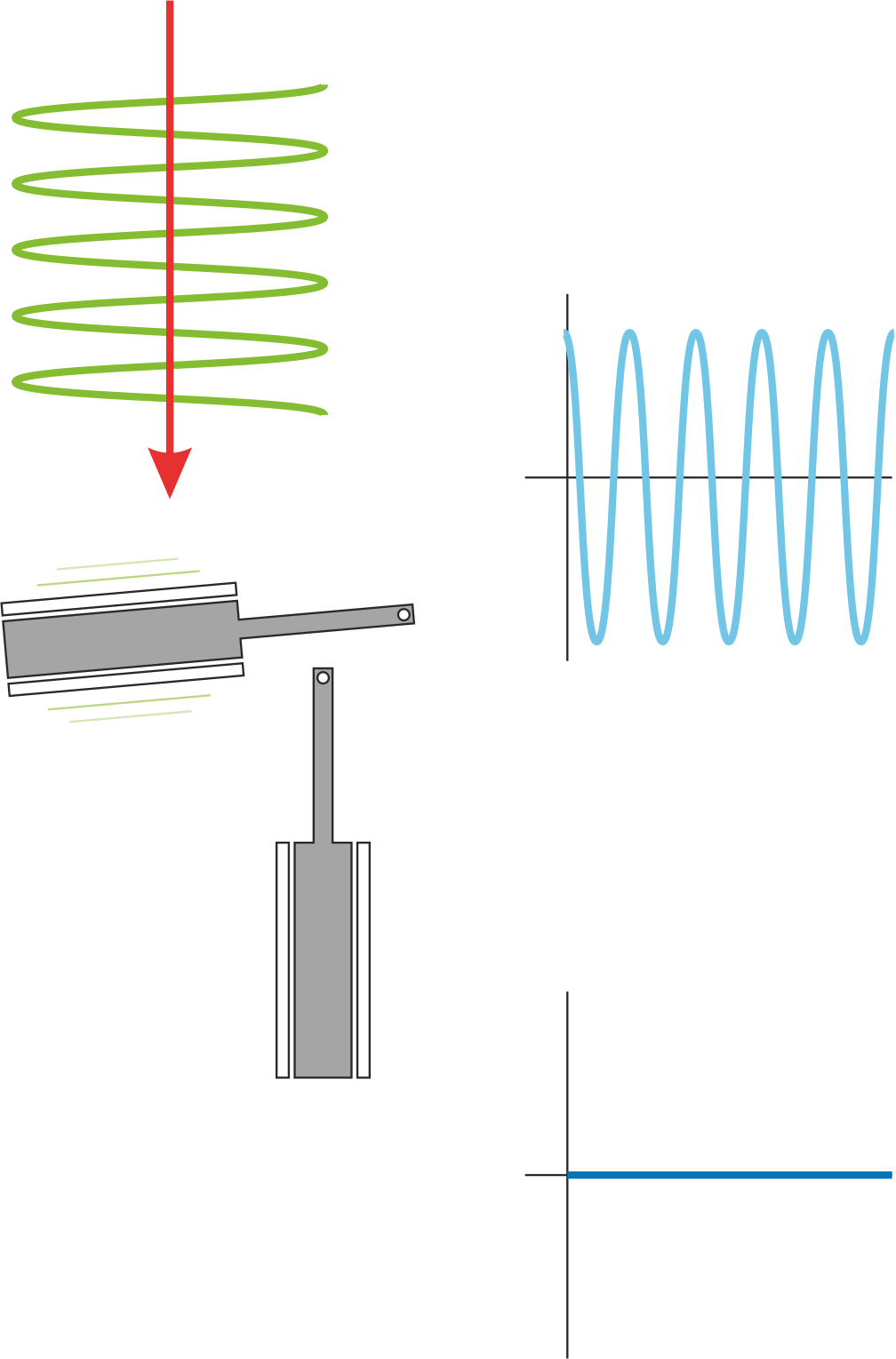If the input signal arrives at 45° to one of the components, the two outputs are no longer equal, as would be the case with an ideal instrument.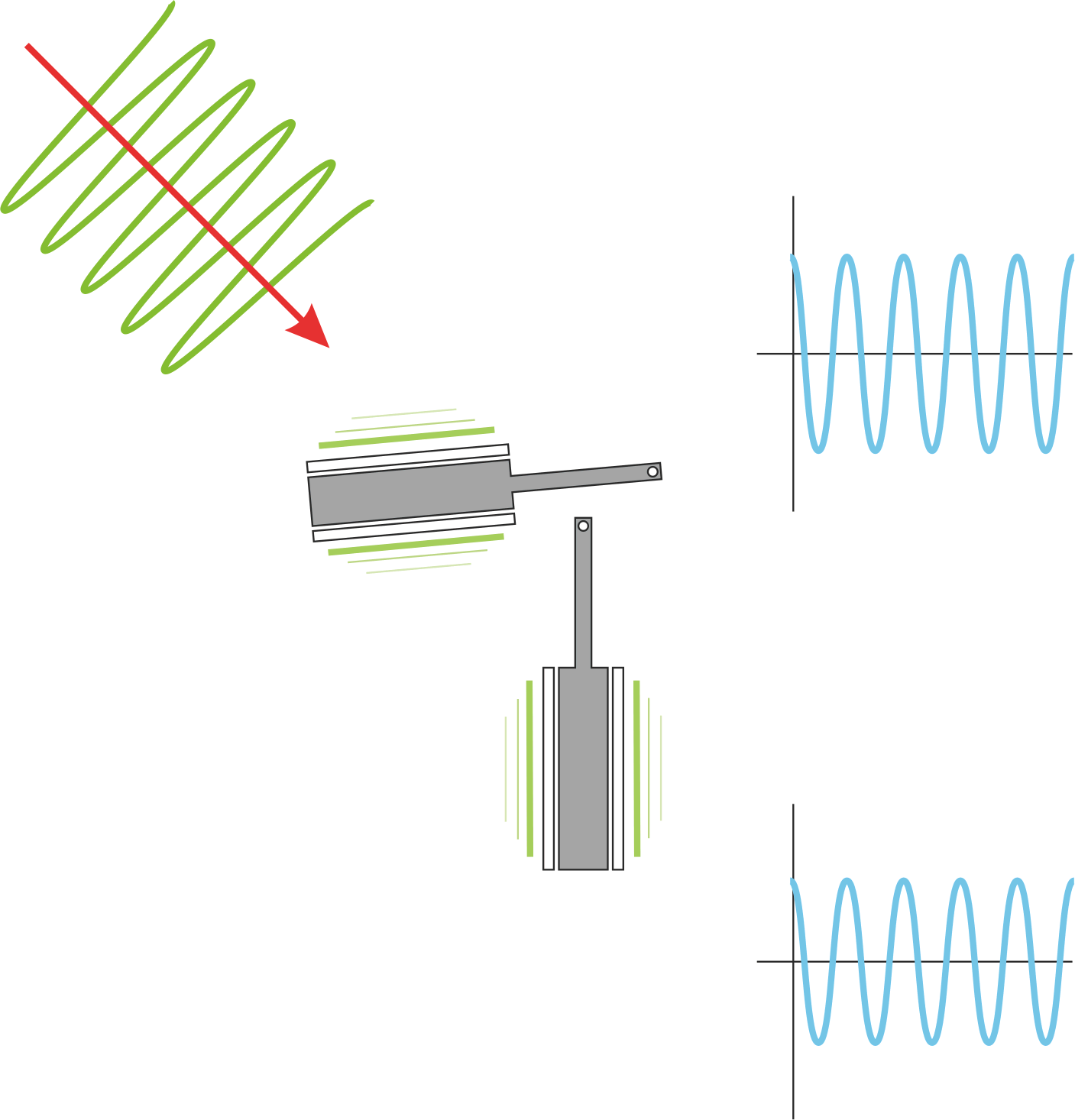To measure the cross-axis rejection ratio for the two horizontal components, we can place the instrument on a shake-table and rotate it until that output at, say, the N/S component is at a maximum. This ensures that the motion of the shake table is perfectly perpendicular to the N/S mass.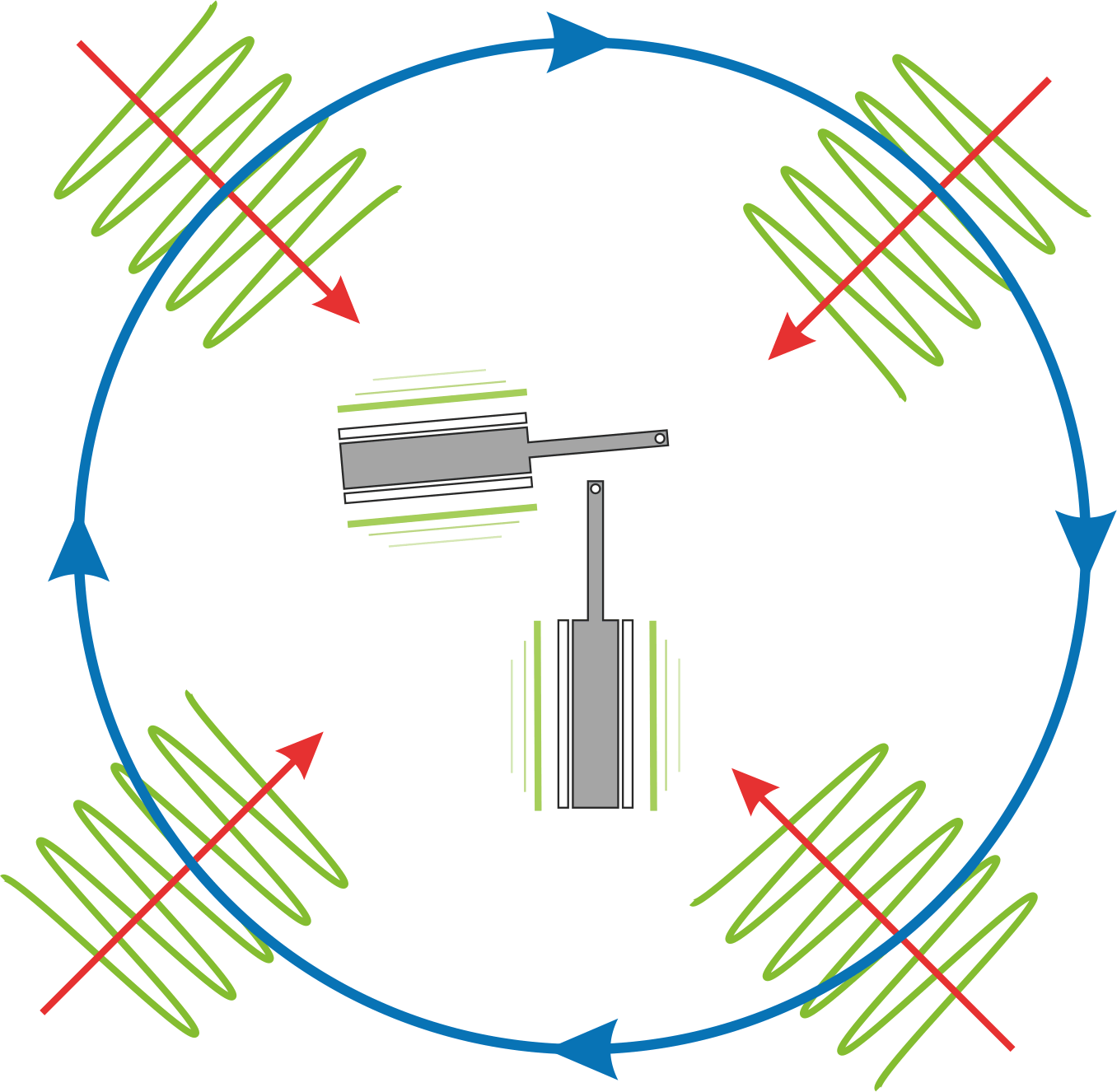We can then measure the amplitude of the output of the E/W channel (AE/W) and divide this by the output at the N/S channel (AN/S). The formula AE/W ÷ AN/S then gives us the cross-axis rejection ratio. Because this fraction is so small, it is normal to express it in deciBels.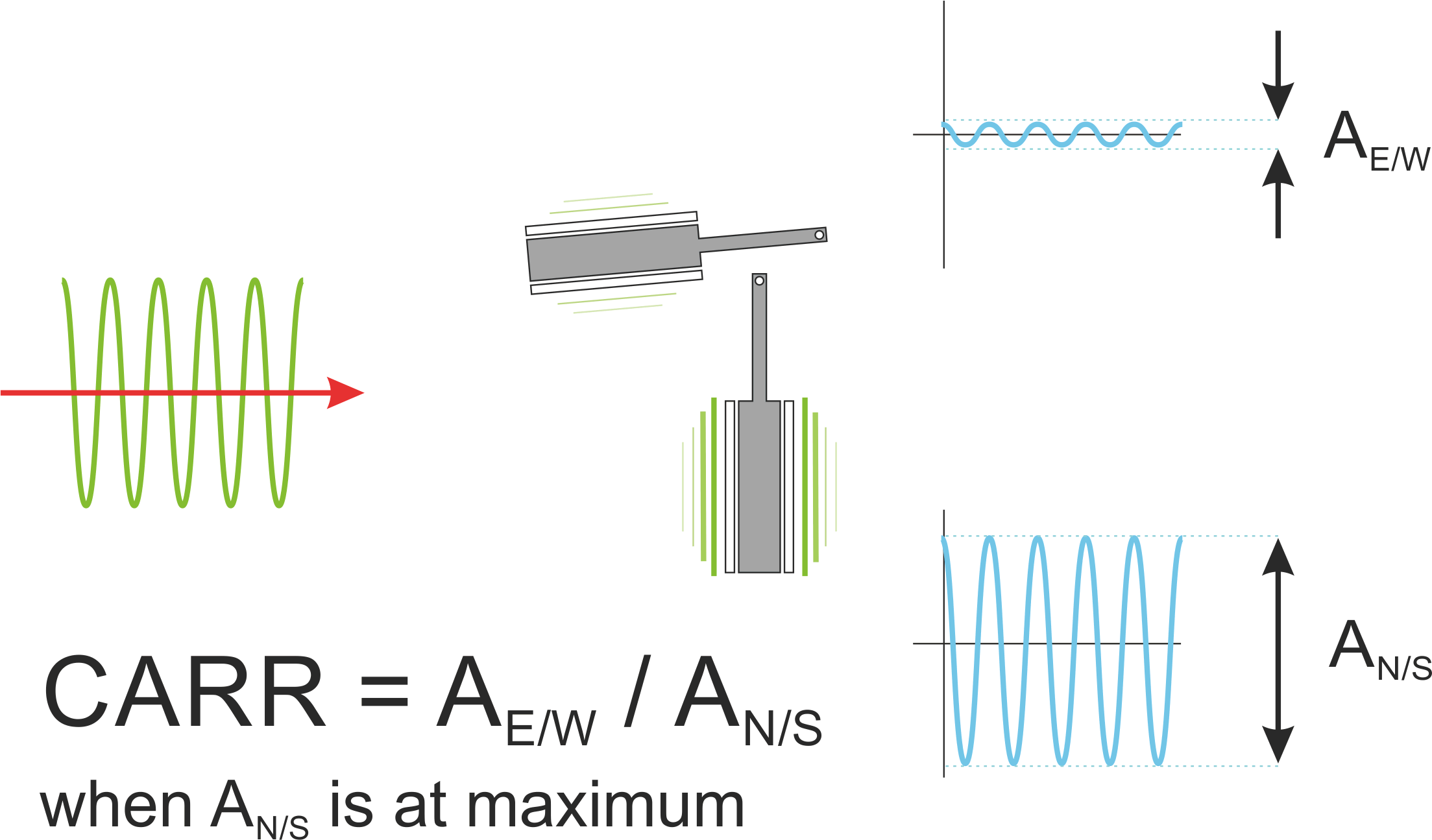Measuring cross-axis rejection ratios between a vertical and a horizontal component is harder but the underlying principles are identical.

Where the cross-axis rejection ratio for a triaxial instrument is given as a single number, this is, by convention, the largest of the three measurable ratios.